pandas python 写入_python pandas 写入 - CSDN

• 一、读取文本格式数据注：索引：将一个或者多个列返回dataframe来处理，获取列名；类型推断和数据转换：用户定义值得转换，缺失值的标记等；日期解析：包括组合功能，比如将分散在多个列中的日期数据组合成单个列；...
一、读取文本格式数据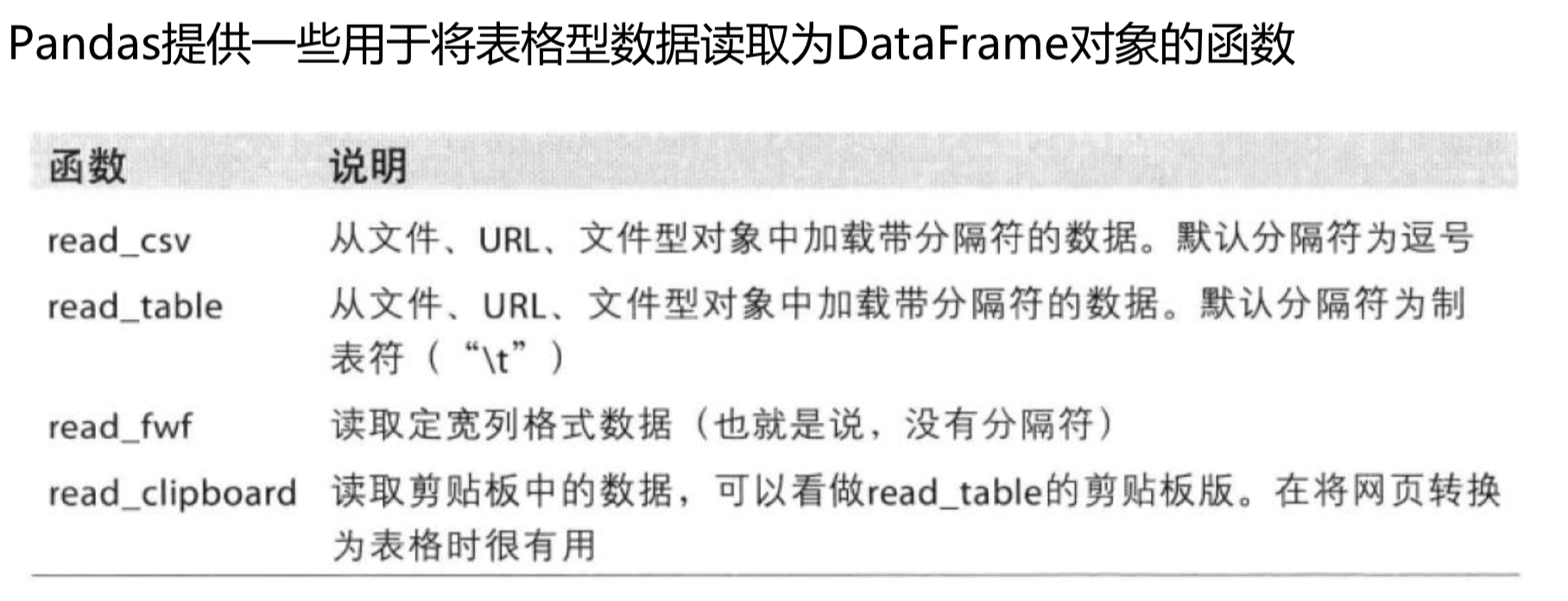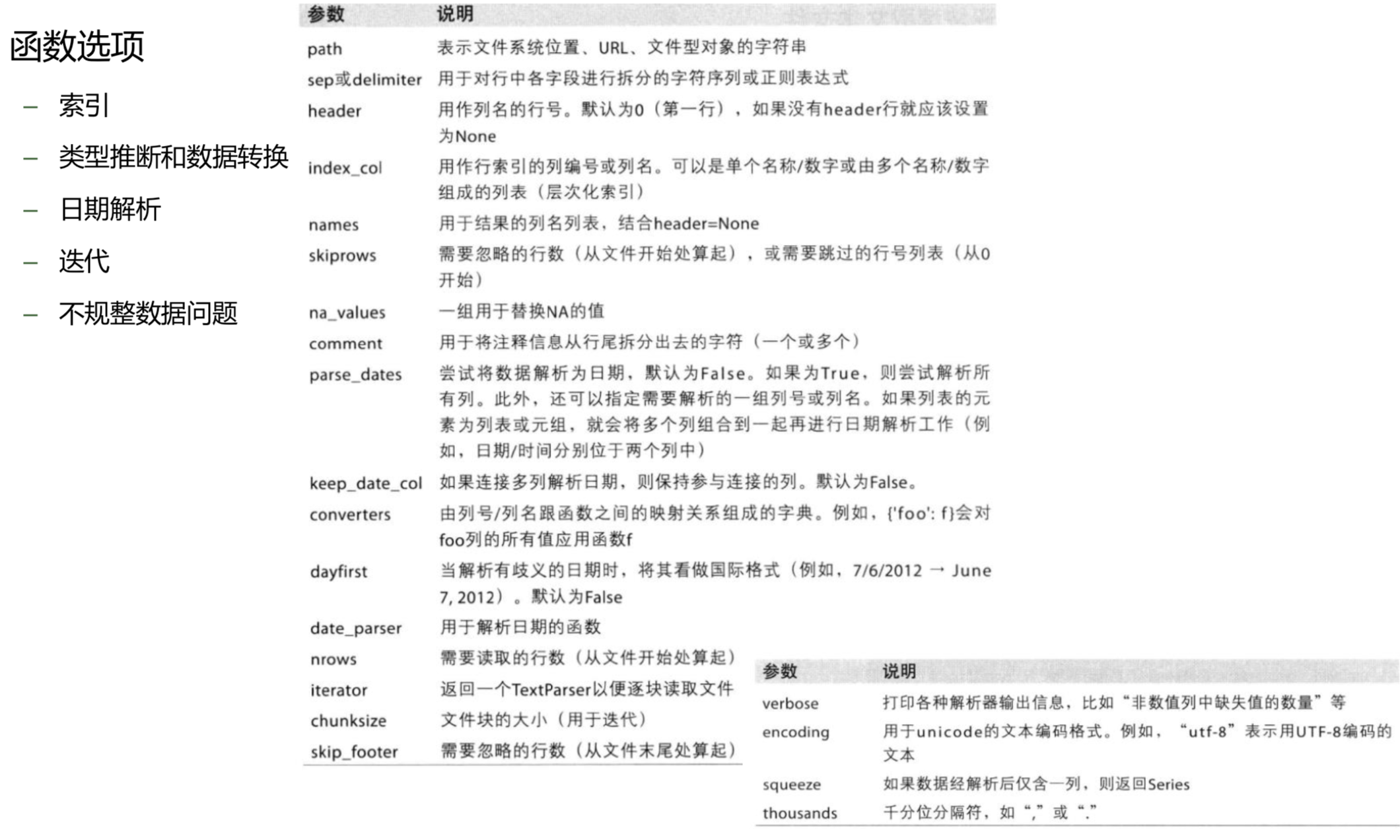注：索引：将一个或者多个列返回dataframe来处理，获取列名；类型推断和数据转换：用户定义值得转换，缺失值的标记等；日期解析：包括组合功能，比如将分散在多个列中的日期数据组合成单个列；迭代：支持大文件逐块迭代，而不是一次性读取；不规整数据问题：可以跳过，清洗。二、示例操作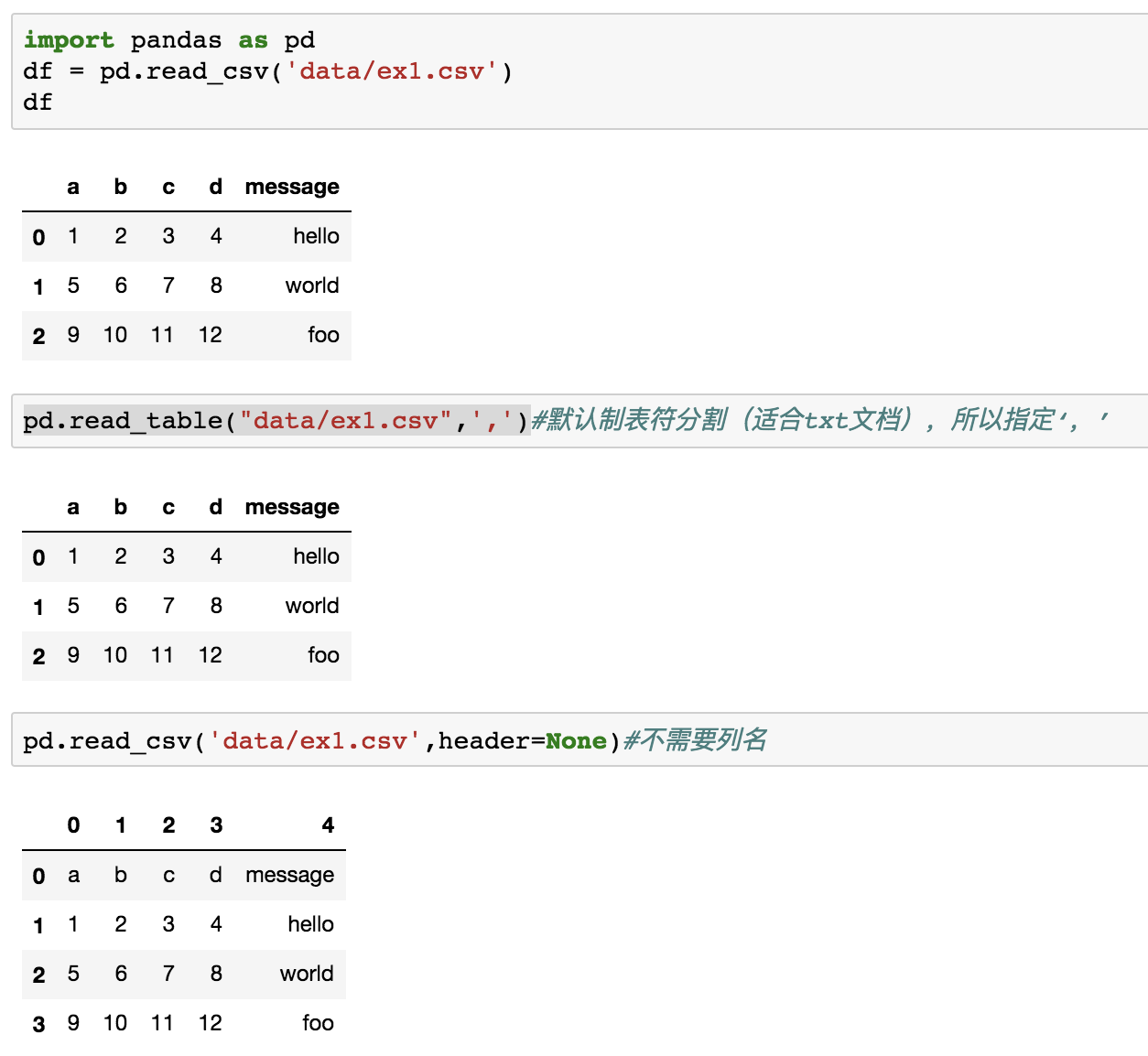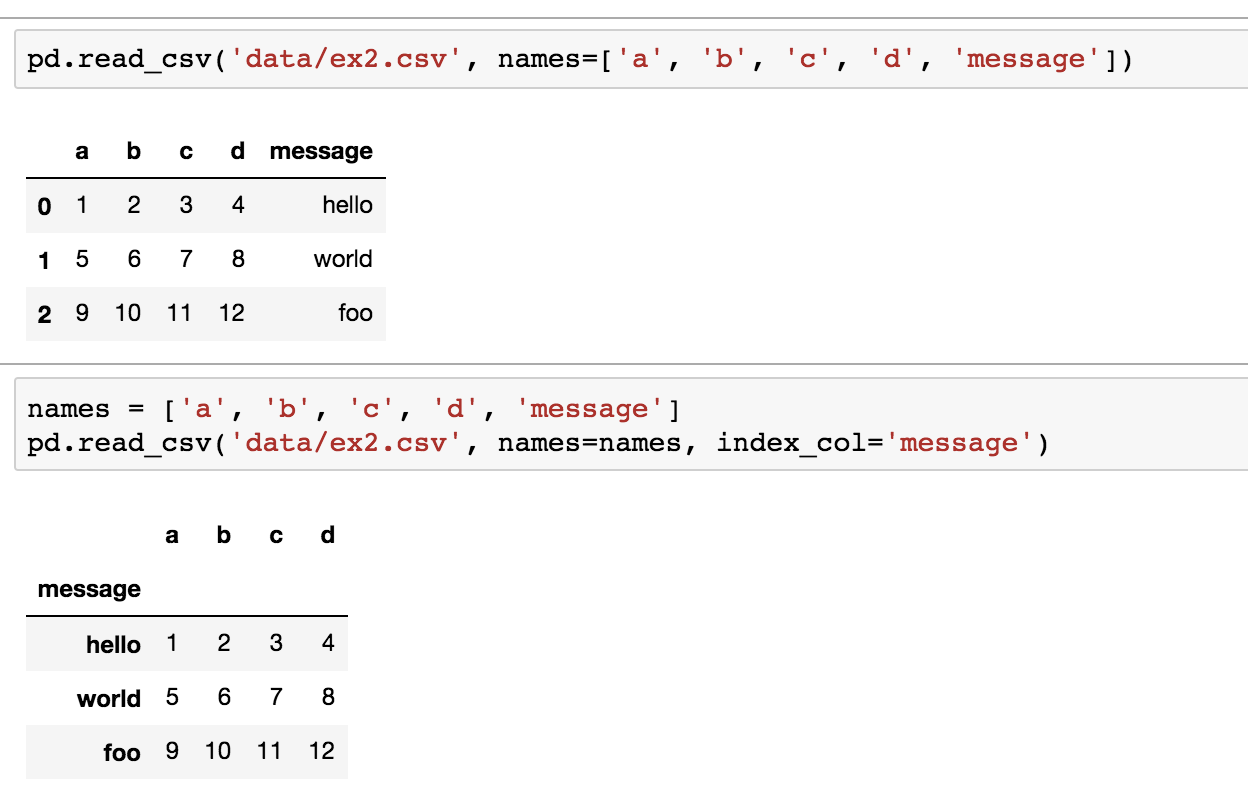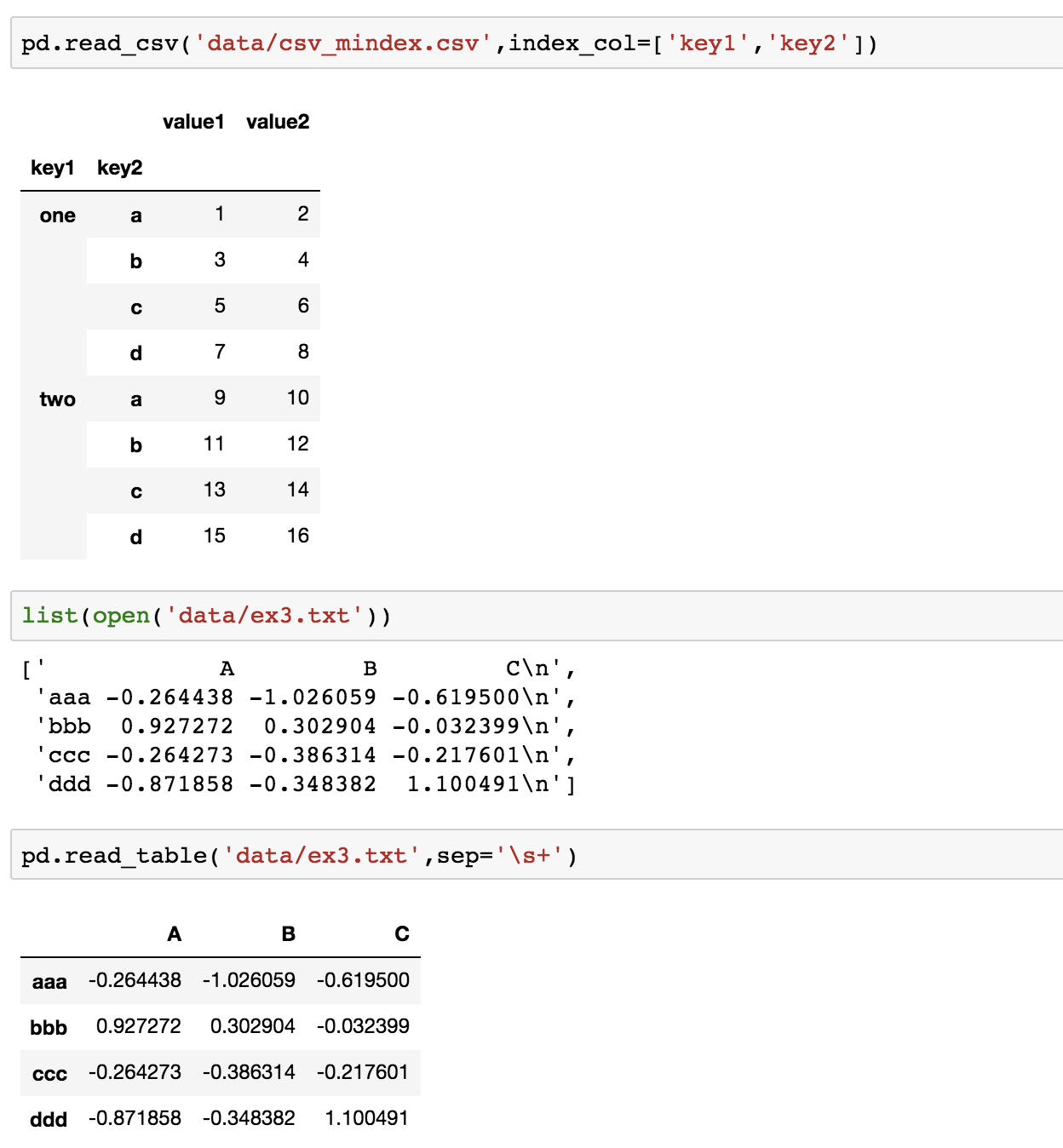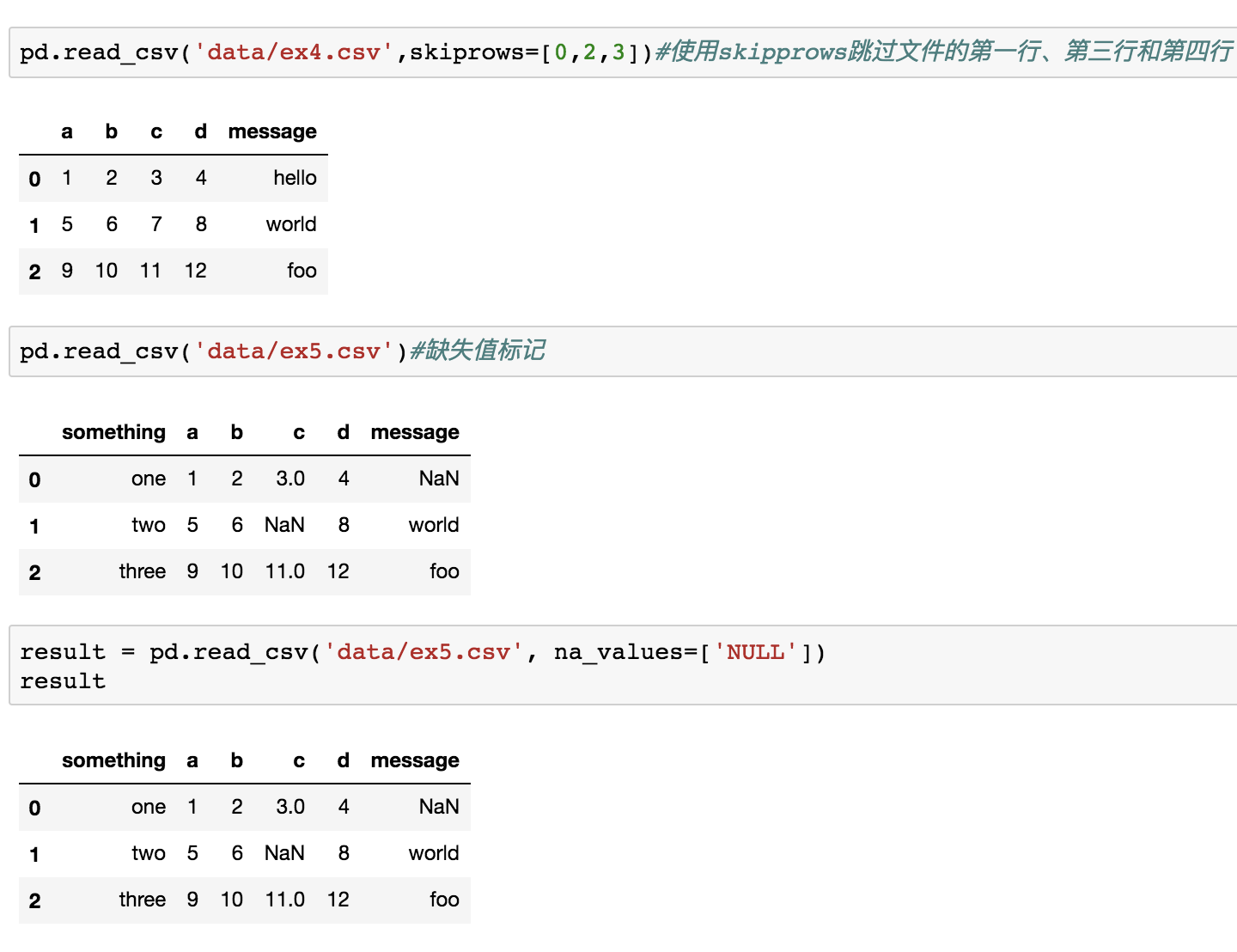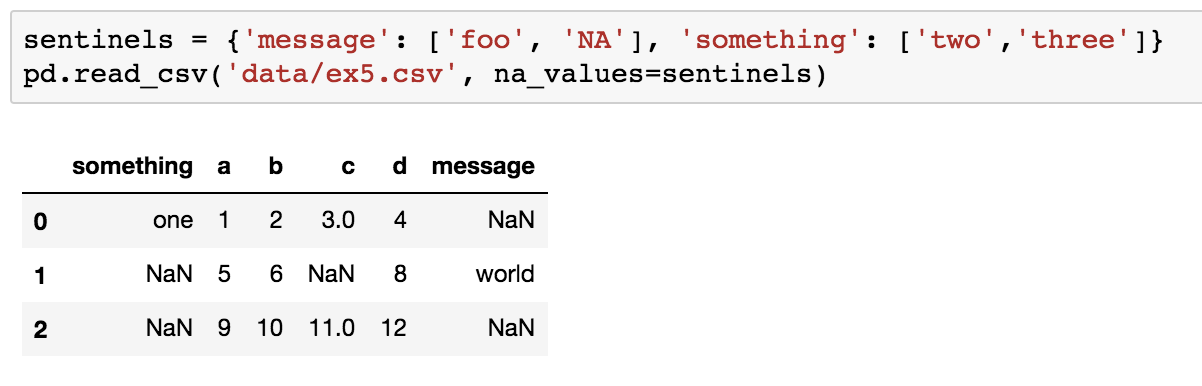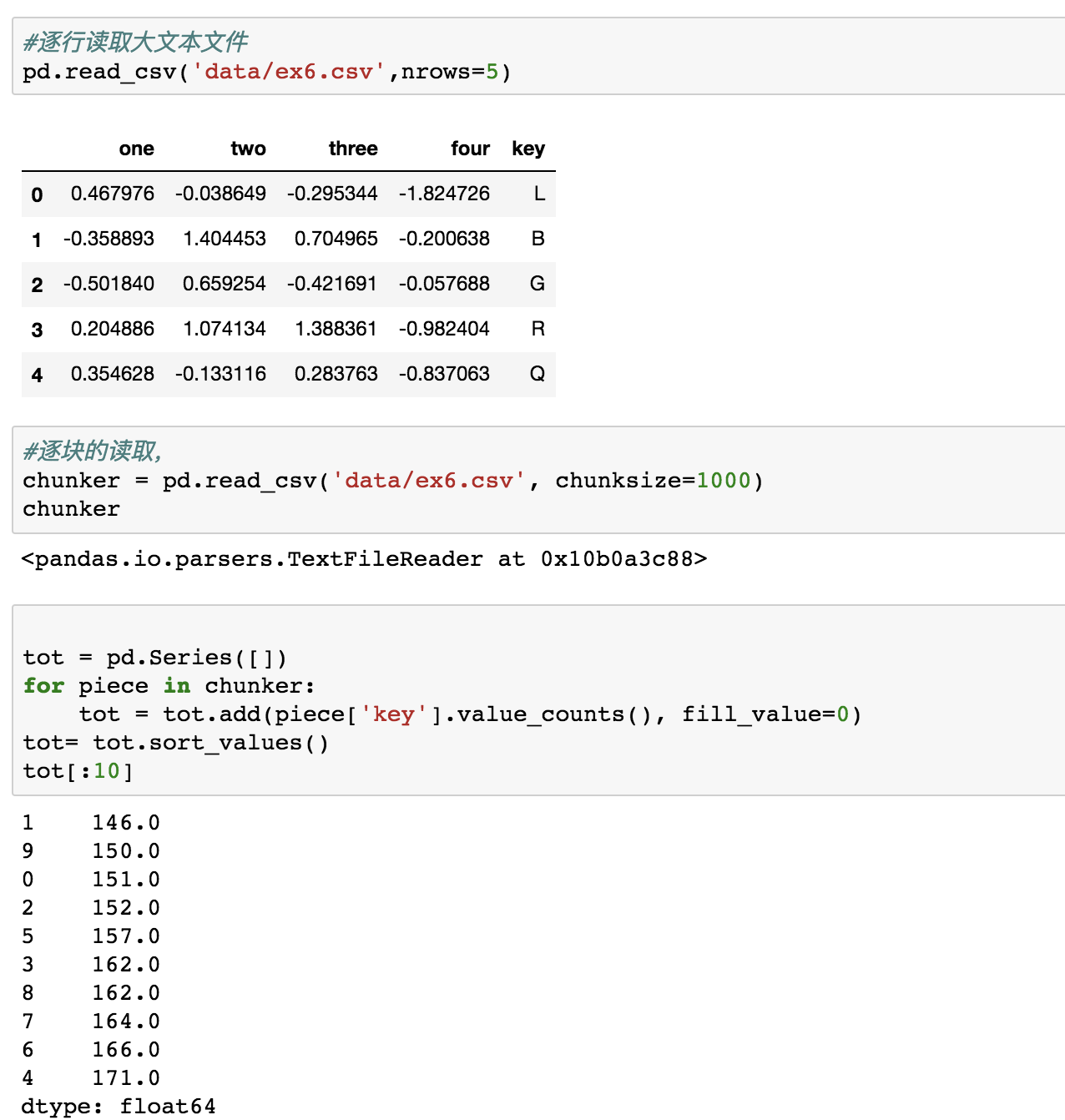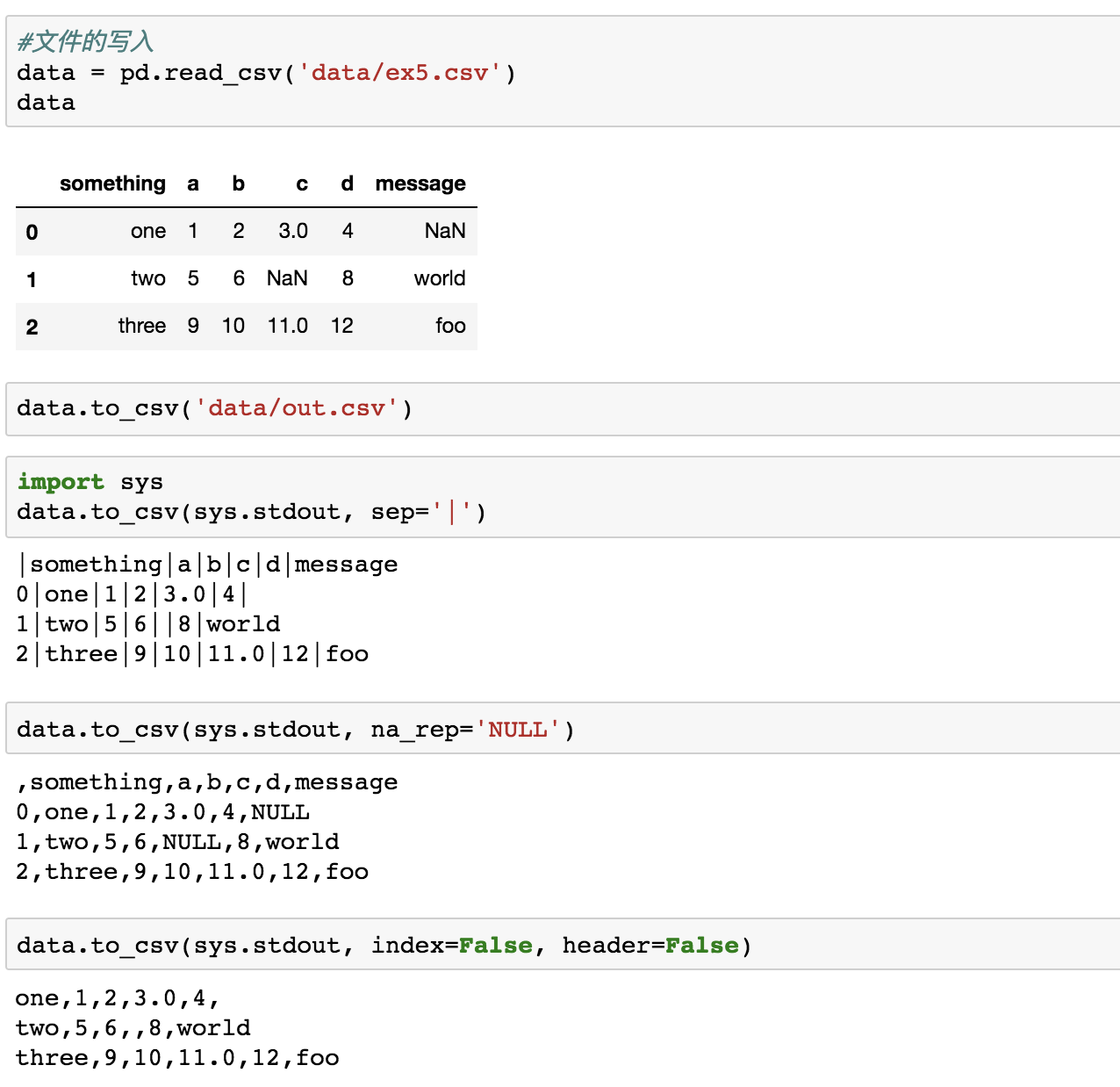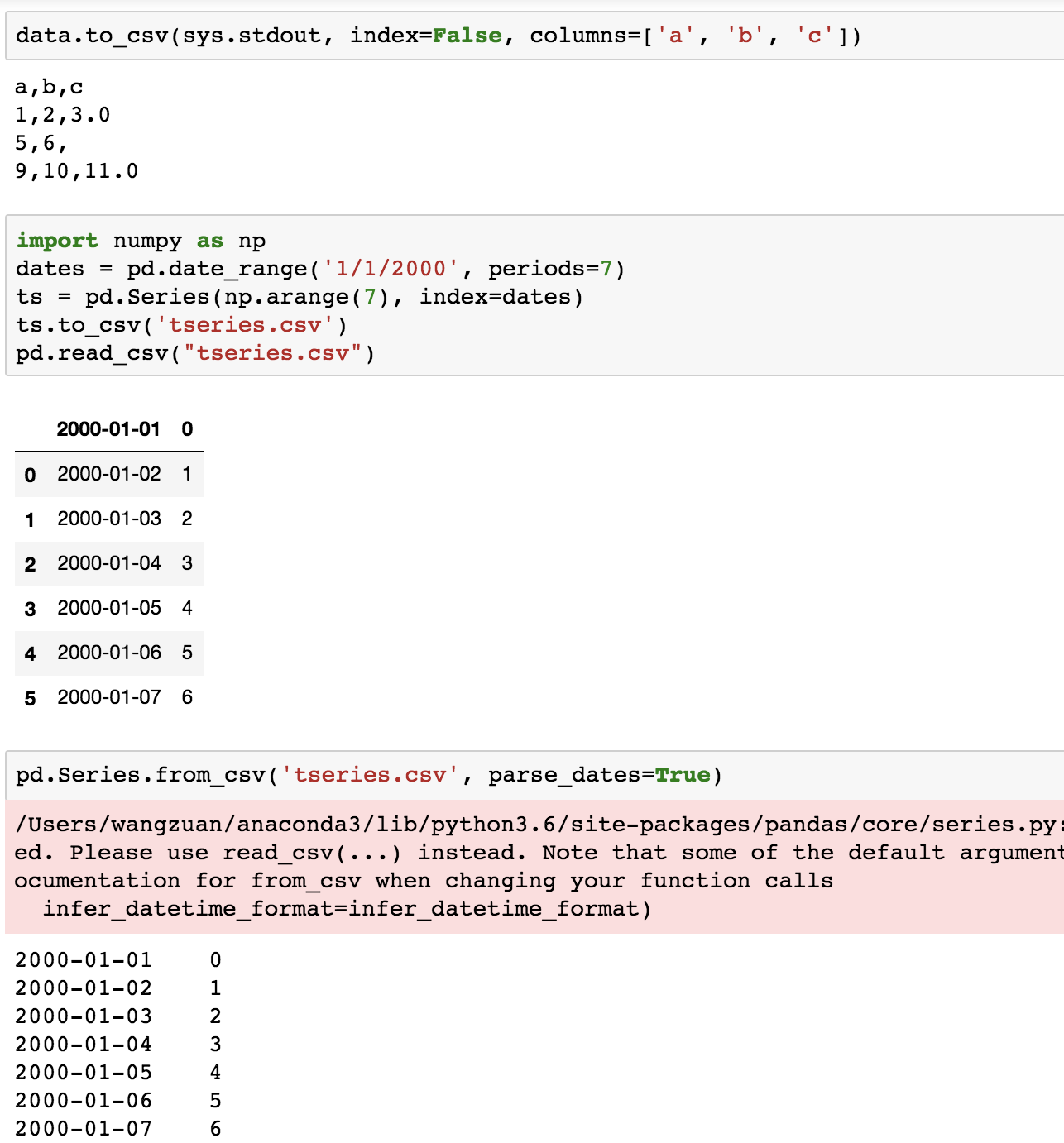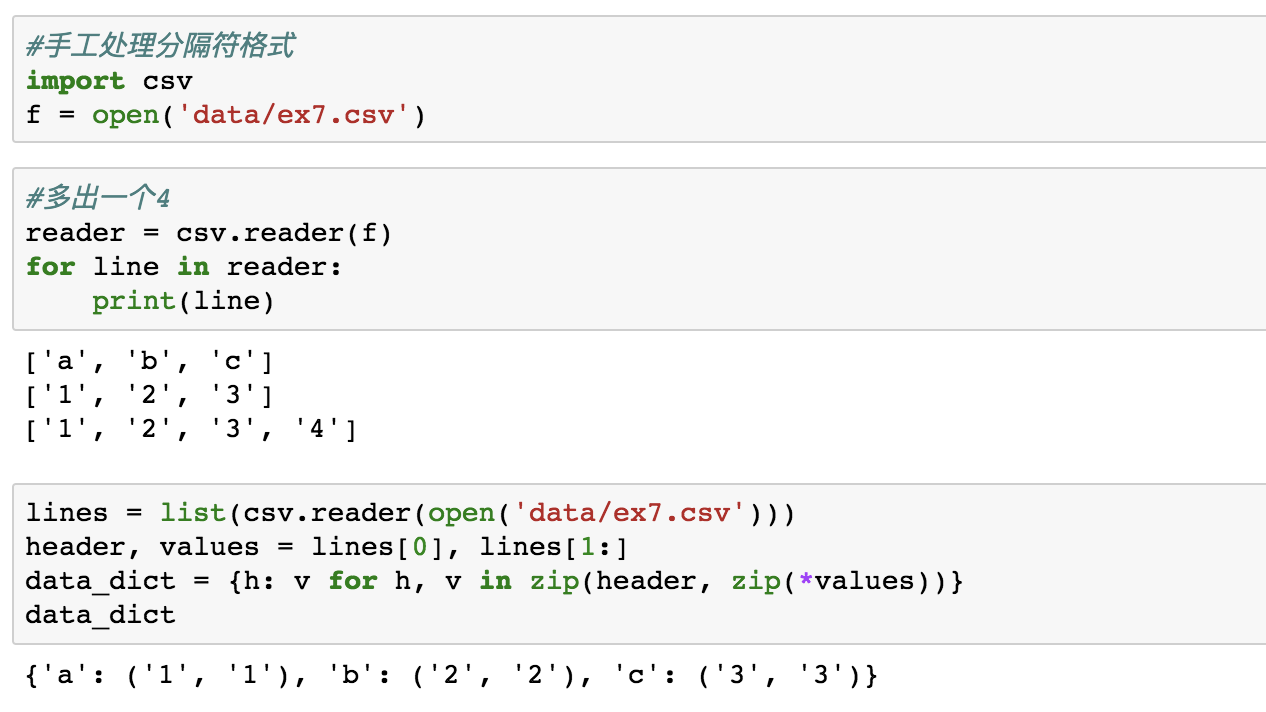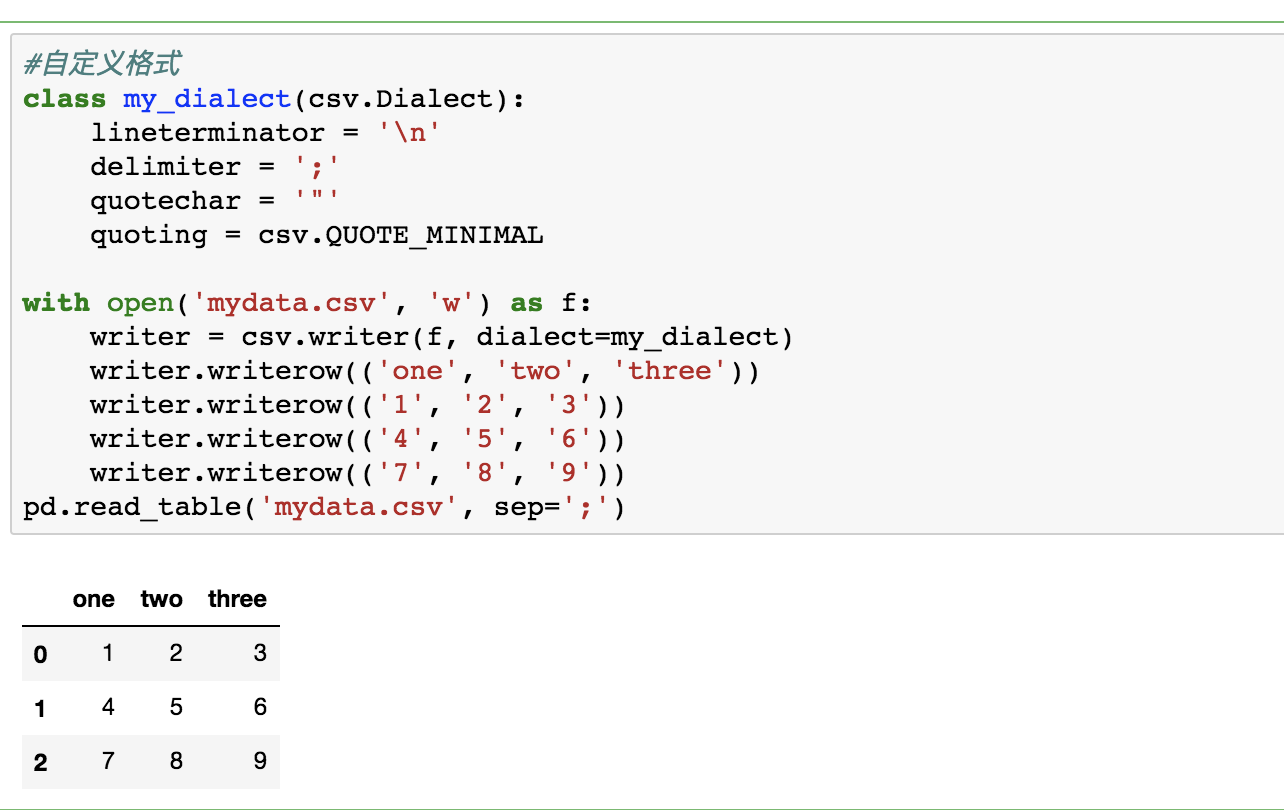展开全文Python Pandas
• ## python写入csv文件的几种方法总结

万次阅读 多人点赞 2017-12-27 20:09:53
最常用的一种方法，利用pandas包import pandas as pd#任意的多组列表 a = [1,2,3] b = [4,5,6] #字典中的key值即为csv中列名 dataframe = pd.DataFrame({'a_name':a,'b_name':b})#将DataFrame存储为csv,index表示...
最常用的一种方法，利用pandas包

import pandas as pd

#任意的多组列表
a = [1,2,3]
b = [4,5,6]

#字典中的key值即为csv中列名
dataframe = pd.DataFrame({'a_name':a,'b_name':b})

#将DataFrame存储为csv,index表示是否显示行名，default=True
dataframe.to_csv("test.csv",index=False,sep=',')

   a_name  b_name
0       1       4
1       2       5
2       3       6

同样pandas也提供简单的读csv方法

import pandas as pd
data = pd.read_csv('test.csv')

会得到一个DataFrame类型的data，不熟悉处理方法可以参考pandas十分钟入门

另一种方法用csv包，一行一行写入

import csv

#python2可以用file替代open
with open("test.csv","w") as csvfile:
writer = csv.writer(csvfile)

#先写入columns_name
writer.writerow(["index","a_name","b_name"])
#写入多行用writerows
writer.writerows([[0,1,3],[1,2,3],[2,3,4]])

index   a_name  b_name
0       1       3
1       2       3
2       3       4

import csv
with open("test.csv","r") as csvfile:
print line

以上。
展开全文python csv
• 1.安装pandas pip install pandas 导入模块并将数据写入csv文件 def write_csv(sql_data): file_name = 'test1.csv' save = pandas.DataFrame(list(sql_data)) try: save.to_csv(file_name) except Unic...
1.安装pandas
pip install pandas


导入模块并将数据写入csv文件

def write_csv(sql_data):
file_name = 'test1.csv'
save = pandas.DataFrame(list(sql_data))
try:
save.to_csv(file_name)
except UnicodeEncodeError:
print("编码错误, 该数据无法写到文件中, 直接忽略该数据")

write_csv(sql_data)

【注】sql_data是你要写如csv文件的数据


展开全文• ## pythonpandas读写excel

千次阅读 2018-07-20 18:07:47
读取excel import pandas as pd df = pd.read_excel('data....写入excel import pandas as pd df = pd.read_excel('data.xlsx') writer = pd.ExcelWriter('out.xlsx') df2 = pd.DataFrame(data = df['colum...

读取excel

import pandas as pd
df = pd.read_excel('data.xlsx')

写入excel

import pandas as pd
writer = pd.ExcelWriter('out.xlsx')
df2 = pd.DataFrame(data = df['column0']) #取出data的column存入out.xlsx中
df2.to_excel(writer, 'sheet1', index = False) #只保存column而没有index
writer.save()
展开全文• 我们越来越多的使用pandas进行数据处理，有时需要向一个已经存在的csv文件写入数据，传统的方法之前我也有些过，向txt，excel文件写入数据，传送门：Python将二维列表（list）的数据输出（TXT，Excel）  pandas to...pandas Python 数据处理 写入文件
• 使用pandas模块中的DtaFrame sep =‘，’即使用分隔符分割需要保存的内容，‘’如果里面不写默认是用” ，“来分割 na_rep='NA'确实值保存为'NA'如果不写默认是空 float_format='%.2f'保留两位小数 原始值 ...pandas
• 重点是同一个sheet！！！ 使用ExcelWriter多次写入同一个excel的多个sheet（保留原内容），这个已经知道如何实现python
• python读写文件有时候会出现 ‘XXX’编码不能打开XXX什么的，用记事本打开要读取的文件，...在pandas中读写csv时候通过制定encoding可以有效防止excel打开或者写入中文乱码 data.to_csv(f_out,index=False,encodipython pandas csv
• 使用pandas操作excel，同时写入数据到多个Sheet def write_excel_pd(file, sheet, data): """ excel title: name / age df1 = { 'name': [1, 2, 3], 'age': [1, 2, 3] } pandas DataFram...python pandas excel
• 导入实验常用的python包。如图2所示。【import pandas as pd】pandas用来做数据处理。【import numpy as np】numpy用来做高维度矩阵运算.【import matplotlib.pyplot as plt】matplotlib用来做数据可视化。3、pandas...
• 先看下需要存入的df数据：安装并导入需要的库：先创建数据库：开始直接一条pandas入库：入库后查看数据：注意：（1）再进行入库的时候，他会根据列自动选择类型，可能为double、float等，后面如果出现类型不匹配，就...
• 如果要对同一个excel 写入多个sheet 的话，普通叠加的方式会覆盖之前写入的sheet. 所以这里提出了一种简单的读写方式。 注意， 这里需要提前安装openpyxl 库， 但是不需要 import pandas as pd df1 = pd.DataFrame({...
• 在使用python处理数据的过程中，经常需要做一些数据读取和写入的工作，比较常用的数据格式是csv，csv文件是一种以逗号分割字符的文件形式 例如：demo.csv，一个很简单的csv文件 name,score alex,1 jon,2 sansa,...Python pandas csv
• Python简单教程:利用pandas对数据的操作(写入excel) 因为之前在对Excel操作的时候，遇到了一些坑。估计小白们都会遇到，所以做了一个最简单的教程，可以为小白避免了很多坑。 代码过于简单，大佬勿喷 材料准备: 1....
• python按照列写入csv文件 可以利用pandas包来按照列写入csv文件： import pandas as pd #a和b的长度必须保持一致，否则报错 a = [x for x in range(5)] b = [x for x in range(5,10)] #字典中的key值即为csv中...
• 将DataFrame中的内容添加到一个已有的excel...import pandas as pd wb = openpyxl.load_workbook('测试.xlsx') #如果有多个模块可以读写excel文件，这里要指定engine，否则可能会报错 writer = pd.ExcelWriter('测...python pandas excel dataframe
• 原文链接：...原文：df.to_csv(‘my_csv.csv’, mode=‘a’, header=False) 试了原文的方式，一直写入失败，然后换成如下方式，即mode=‘a+’,而不是mode=‘a’ df.to_csv(‘my_csv.csv’, mode=‘a+’,...
• python pandas 读写文件： 读： import pandas as pd file_path = "abc.csv" data = pd.read_csv(file_path) # data 是个dataframe,可以进行 dataframe 的操作（切割，分块，计算等等） 写： data =...python pandas
• import pandas as pd pd.DataFrame(shop_pay_count).to_csv('shop_pay_count_demo.csv') DataFrame 生成的是一个新的对象，示例2： import pandas as pd inp = [{'c1':10, 'c2':100}, {'c1':11,'c2':110}, {'c1...python pandas 数据操作
• 本文实例讲述了Python3使用pandas模块读写excel操作。分享给大家供大家参考，具体如下： 前言 Python Data Analysis Library 或 pandas 是基于NumPy 的一种工具，该工具是为了解决数据分析任务而创建的。Pandas ...python
...